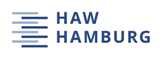# Mach number, relative thickness, sweep and lift coefficient of the wing - An empirical investigation of parameters and equations

Author: Simona Ciornei

Project

## Abstract

12 equations were investigated to calculate the relative thickness t/c of the wing of an aircraft. The calculated relative thickness was taken as the average relative thickness of the wing. The data obtained from these 12 equations was checked against the given average relative thickness of 29 carefully selected aircraft, spanning a space of the parameters Mach number, lift coefficient, sweep, and type of airfoil. Some equations selected are empirical in their nature (partly based on aerodynamic derivation) other equations are purely statistical. Whenever equations had free parameters, these were optimized against the aircraft data. The best equation turned out to be an equation based on nonlinear regression. It achieved a Standard Error of Estimate of only 0.75 % for the average relative thickness of the wing. Torenbeek’s equation will probably be preferred by those that like to see an equation that is based on aerodynamic considerations. It achieved a Standard Error of Estimate of 0.80 % when all its free parameters were considered for optimization. The worst equation produced an Standard Error of Estimate of 8 %. For an airfoil with 10 % relative thickness this would give an unacceptable 10 % +/- 8 % mean band of values for t/c which renders equations like this quite useless.